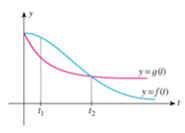Chapter 2.6, Problem 7E

Chapter
Section
Textbook Problem

Effect of a Bactericide on Bacteria In the following figure, f(t) gives the population P1 of a certain bacteria culture at time t after a portion of Bactericide A was introduced into the population at t = 0. The graph of g gives the population P2 of a similar bacteria culture at time t after a portion of Bactericide B was introduced into the population at t = 0. a. Which population is decreasing faster at t1? b. Which population is decreasing faster at t2?c. Which bactericide is more effective in reducing the population of bacteria in the short run? In the long run?

(a)

To determine

The population which decreases faster at t1 .

Explanation

Given information:

The bacteria population P1 and P2 are shown in the given figure by y=f(t) and y=g(t) respectively, where population P1 shows the effect of Bactericide A and population P2 shows the effect of Bactericide B.

Figure (1)

Calculation:

From Figure (1), it is noticed that the x and y-axis represent the time and population of bacteria respectively. The blue and pink curve represents the population P1 and P2 after the effect of Bactericide A and Bactericide B respectively.

Since the rate of change of population represent the rate of increase and rate of decrease in the population, therefore the slopes of the tangent line at any point shows the increasing or decreasing rate at that point of time.

Draw the tangent lines on the curves y=f(t) and y=g(t) at point t1 to compare the slopes of the tangents at point t1

(b)

To determine

The population which decreases faster at t2 .

(c)

To determine

The bactericide which is more effective in short run and the bactericide that is more effective in long run.

Still sussing out bartleby?

Check out a sample textbook solution.

See a sample solution

The Solution to Your Study Problems

Bartleby provides explanations to thousands of textbook problems written by our experts, many with advanced degrees!

Get Started

Convert the expressions in Exercises 6584 to power form. 3

Finite Mathematics and Applied Calculus (MindTap Course List)

Find the mean for the scores in the following frequency distribution table: X f 6 1 5 4 4 2 3 2 2 1

Essentials of Statistics for The Behavioral Sciences (MindTap Course List)

In problems 23-58, perform the indicated operations and simplify. 52.

Mathematical Applications for the Management, Life, and Social Sciences

If f(x) = 2e3x, f(x) = a) 2e3x b) 2xe3x c) 6e3x d) 6xe3x

Study Guide for Stewart's Single Variable Calculus: Early Transcendentals, 8th

Self Check Rationalize the numerator: 4+h-2h

College Algebra (MindTap Course List)

Identify possible sources and use them to identify a topic area for research.

Research Methods for the Behavioral Sciences (MindTap Course List)